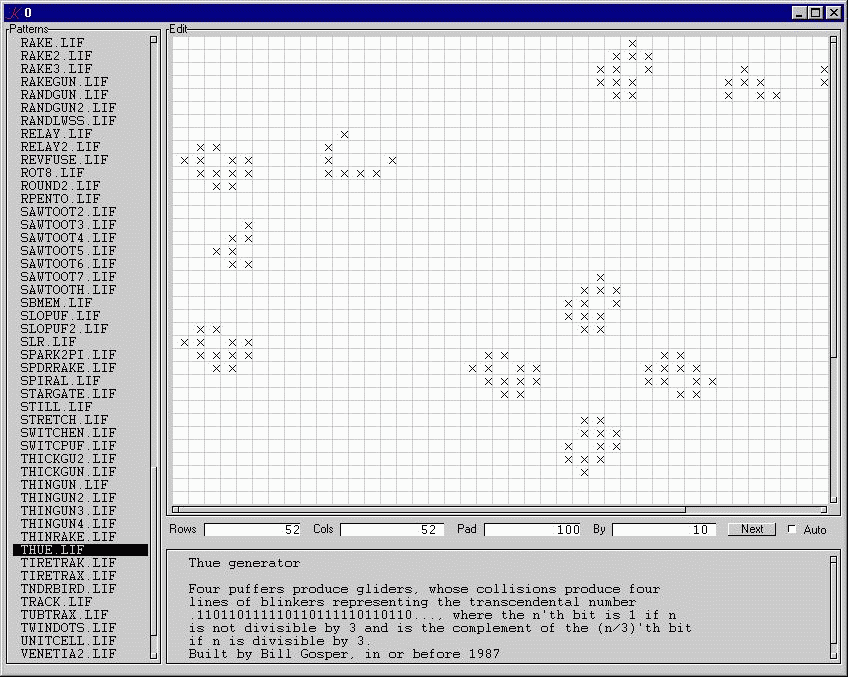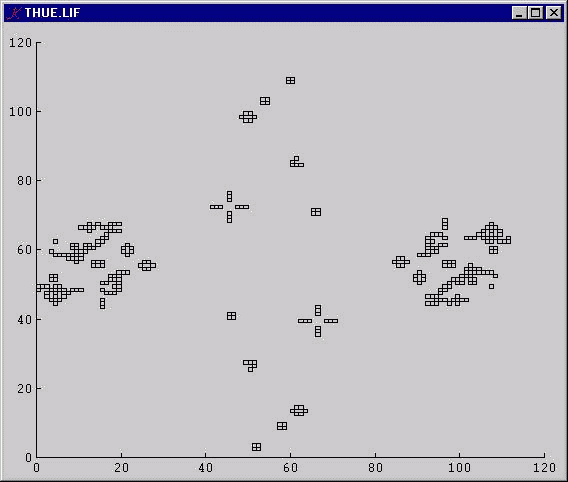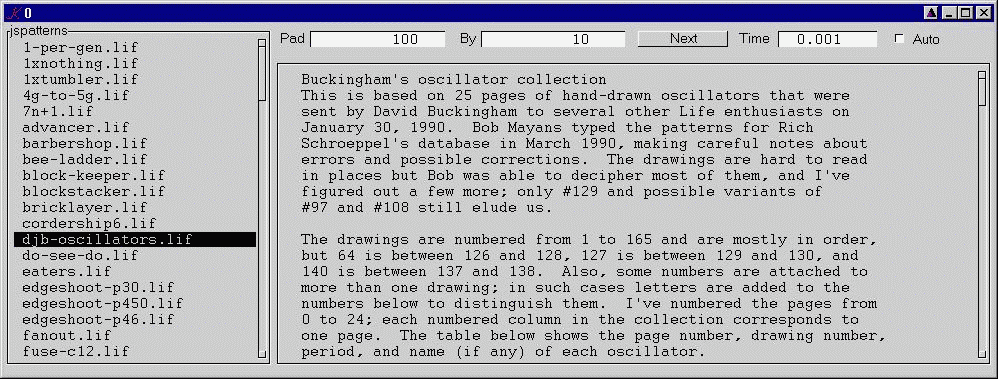# Life in K

A K implementation of the classic APL design contains alternate algorithms for computing the next state of the universe.

```next:{(x&2=k)|3=k:+/adj x}
```next:{s#(v&2=k)|3=k:+/adj[v:,/x]. s:^x}
index:{(x+/:(1;-1;z;-z;z+1;z-1;-z+1;-z-1))!y*z}```

The first algorithm takes the current state (a boolean matrix), performs eight shifts to align cell x[i;j] with its eight neighbors, then sums the matrices and applies the game rule.

The second algorithm first converts the matrix to a vector v (v:,/x), then aligns each cell with its neighbors by performing a simple arithmetic calculation on the index-vector !#v.

A much faster method (but see below) uses a vector of indices of the 'on' cells:

```next:{[u;v;s]
a:index[u]. s
w:(?,/a)_dvl u
b:index[w]. s
v:@[v;u;:;1]
(u@&(2=k)|3=k:+/v a),w@&3=+/v b}

index:{(x+/:(1;-1;z;-z;z+1;z-1;-z+1;-z-1))!y*z}```

The 'next' function takes u, a vector of the indices of the 'on' cells, v, a vector of zeros, and s, the shape of the conceptual universe. 'next' computes a, the indices of the neighbors of each 'on' cell, and b, the neighbors of the adjoining zero cells. This approach copes well with very large, very sparse universes.

Two very nice solutions from Swee Heng (Thanks Attila!): here and here.

Arthur's solution:

`f:{3=s-x&4=s:2{+(0+':x)+1_ x,0}/x}`

There are two windows.

The Editor:The Universe:The editor consumes space and time on large patterns, so here is a version which performs all calculations directly on vectors. This version also knows how to read run-length-encoded .lif files and allows the operator to set the time-interval for 'auto' mode:(Thanks Mike!)

To run, make sure you have at least one directory of life-patterns* (here's the one life.k defaults to). Then say:

`k life`

or

`k life path-to-pattern-directory`

Coming soon: parallel life.

*I've included two life-pattern directories: here and here.

This version of Life has a long history in the APL/J/K community.

The array algorithm for computing next generation has been rediscovered independently several times -- it is that obvious. My version (for VS APL) dates from around 1980.

The GUI is based on one devised by Mike Rosenberg, and first appeared on the K mailing list in 1999.